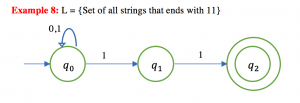Example of Conversion of Epsilon ε-NFA to NFA – 3

Example 3:  Convert the following ε-NFA to its equivalent NFA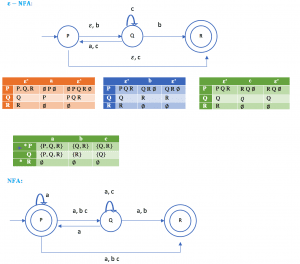Example of Conversion of Epsilon ε-NFA to NFA – 2

Example : Convert the following ε-NFA to its equivalent NFA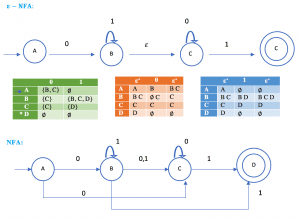Example of Conversion of Epsilon NFA to NFA – 1

Example : Convert the following ε-NFA to its equivalent NFA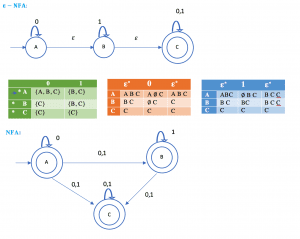Example of Minimization of Deterministic Finite Automata (DFA)

Minimization of DFA (Table Filling Method or Myhill-Nerode Theorem)

Steps:

1. Draw a table for all pairs of states (P, Q)
2. Mark all pairs where Pϵ F and Q∉F
3. If there are any Unmarked pairs (P, Q) such that [δ(P, x),δ(Q, x)] is marked, then mark [P, Q] where ‘x’ is an input symbol. Repeat this until no more marking can be made.
4. Combine all the unmarked pairs and make them a single state in the minimized DFA.

Example: Minimize the following DFA using Table Filling Method.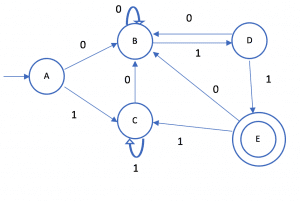Example of Conversion of Non-Deterministic Finite Automata (NFA) to Deterministic Finite Automata (DFA) – 5

Example : Design a NFA for a language that accepts all string over {0,1}, in which the second last symbol is always 1. Then convert it to its equivalent DFA.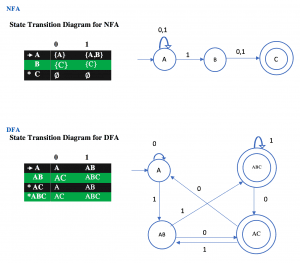Example of Conversion of Non-Deterministic Finite Automata (NFA) to Deterministic Finite Automata (DFA) – 4

Example :Given below is the NFA for a language L= {Set of all string over (0, 1) that ends with 01 }  construct its equivalent DFA.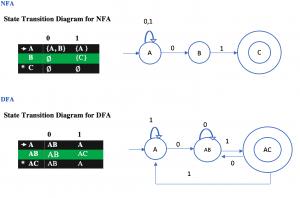Example of Conversion of Non-Deterministic Finite Automata (NFA) to Deterministic Finite Automata (DFA) – 3

Example:  Find the equivalent DFA for the given by M= [{A, B, C}, (a, b), , A, {C}] where δ is given by: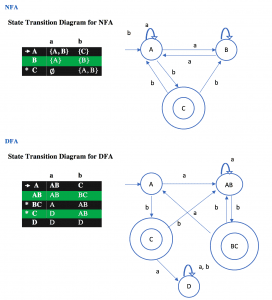Example of Conversion of Non-Deterministic Finite Automata (NFA) to Deterministic Finite Automata (DFA) – 2

Example :  L = {Set of all string over (0,1) that ends with 1}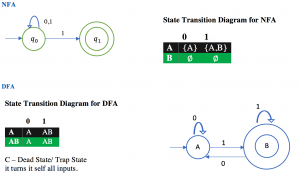Example of Conversion of Non-Deterministic Finite Automata (NFA) to Deterministic Finite Automata (DFA) – 1

Example:  L= {Set of all strings over (0,1) that start with 0}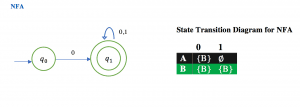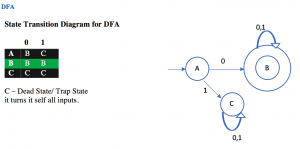Example of Non-Deterministic Finite Automata (NFA) – 8